Get instant live expert help with Excel or Google Sheets“My Excelchat expert helped me in less than 20 minutes, saving me what would have been 5 hours of work!”

#### Post your problem and you’ll get expert help in seconds.

Your message must be at least 40 characters
Our professional experts are available now. Your privacy is guaranteed.

# Learn How to Create a Dynamic Pivot Table in Excel

We can create a dynamic Pivot Table in Excel by copying and pasting a new set of data directly below the data table. Afterward, we will refresh the Pivot Table to include the new data. This is better than the OFFSET function used in earlier Excel versions. The steps below will walk through the process.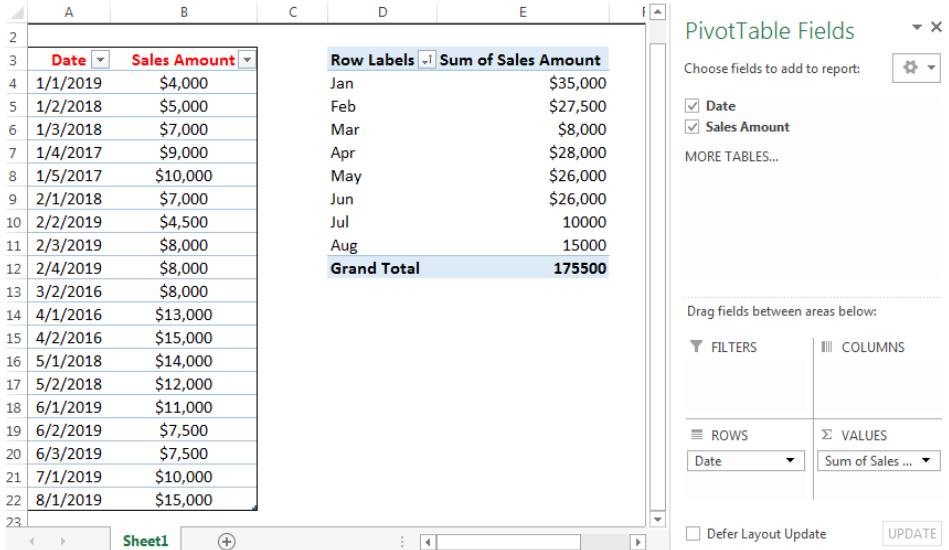Figure 1- How to Create a Dynamic Pivot Table in Excel

## Setting up the Data

• We will use the created Pivot Table in figure 2 to make a Dynamic range Pivot Table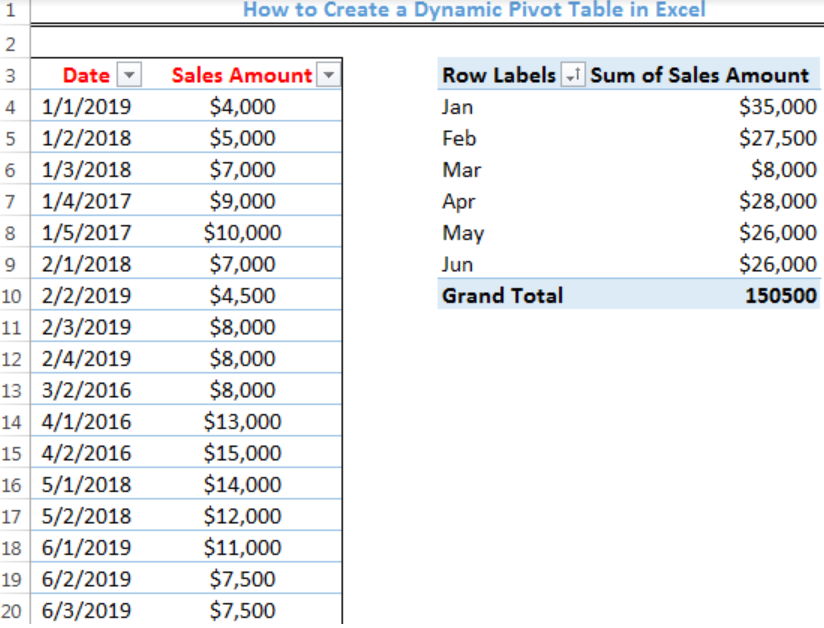Figure 2 – Setting up the Data

## Creation of a Dynamic Pivot Table

• We will add two more dates and sales amount to the data table
• We will do this by clicking Cell A21 and paste the data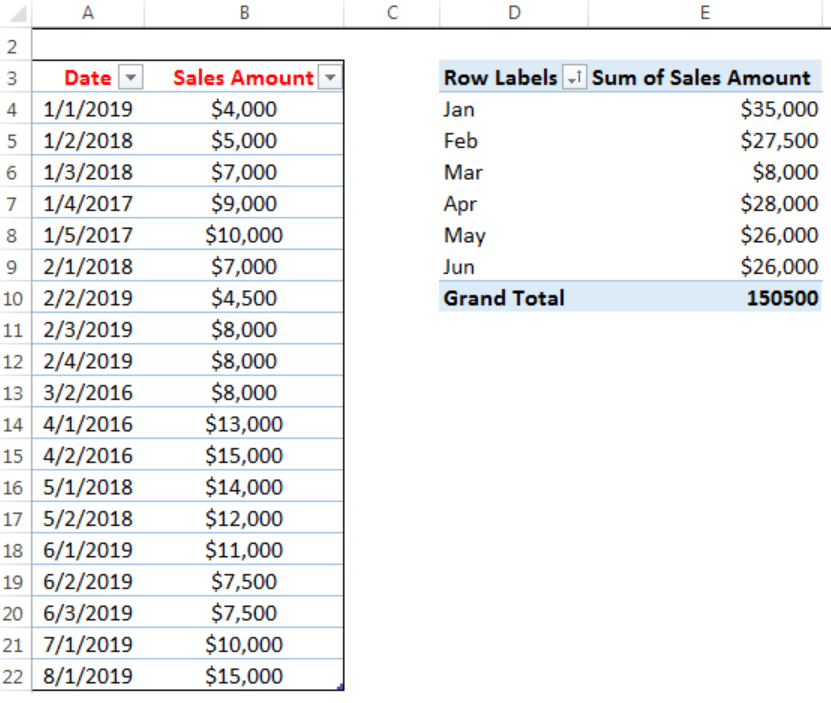Figure 3- Dara added to Row 21 and Row 22

• We will right-click on any part of the Pivot table and select refresh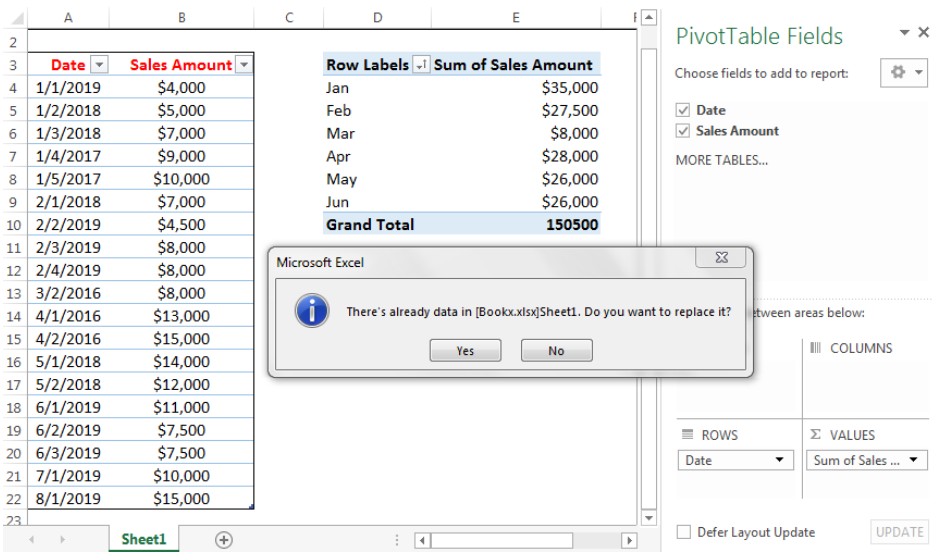Figure 4- Prompt to replace the current Pivot Table

• We will click Yes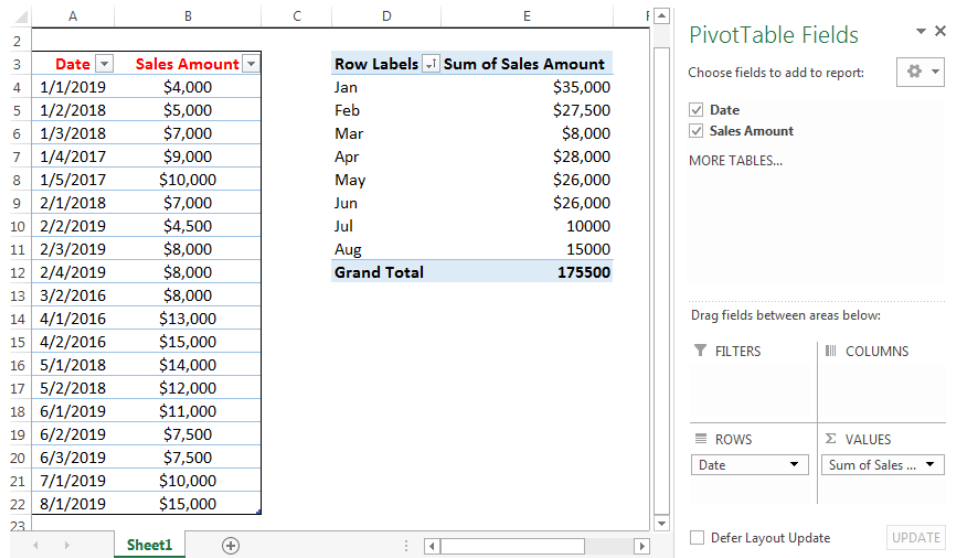Figure 5- Created Dynamic Pivot Table

## Instant Connection to an Expert through our Excelchat Service

Most of the time, the problem you will need to solve will be more complex than a simple application of a formula or function. If you want to save hours of research and frustration, try our live Excelchat service! Our Excel Experts are available 24/7 to answer any Excel question you may have. We guarantee a connection within 30 seconds and a customized solution within 20 minutes.

Solution examplesI need help fixing a Formula on Sheet 2, starting in Column G Row 22. In short, the formula should average the last six dollar amounts unless one of the last 6 dollar amounts is blank or 0. G56 on the same sheet works with the same logic but for only 3 months.
Solved by B. L. in 41 minsHello! There is a column with a certain number of rows in it. I need to calculate two averages: the average of every second row starting from the 1st one (aka the average of every odd-th cell) and the average of every second row starting from the 2nd one (aka the average of every even-th cell). I got tangled up with =OFFSET... Thanks!
Solved by C. J. in 37 minsI'm trying to use offset in a match function. My table is pulling data from a different tab called "operator runs". I want my first row to look at data in B1:B104 for "sample-A" and match "BA" from C1:C104 to pull data from column D. My second row, I want to look up text from F1:F104 and match "BA" from G1:G104 and pull data from column H and so on, offset by 4 columns to the right each time. This is my current function. I don't know how to implement the offset function in this complicated function. =MATCH(1,('Operator runs'!\$B\$1:\$B\$104="Sample-A")*('Operator runs'!\$C\$1:\$C\$104="BA"),0)
Solved by O. L. in 40 minsI'm looking to offset from =today(), for example: If cell A13=today(), display cell A20. If cell B13=today(), display cell B20. If cell C13=today(), display cell C20. I want to do this for 365 rows. Is there a simpler way of doing this than a huge nested IF statement?
Solved by K. H. in 34 minsHi, I need a formula to work out how many values are less than a certain time value, simple "countif" do not work as it is a filtered spreadsheet. Here's the formula that I have got so far: SUMPRODUCT(SUBTOTAL(3,OFFSET(Current_Month!N\$2:N\$10000,ROW(Current_Month!N\$2:N\$10000)-MIN(ROW(Current_Month!N\$2:N\$10000)),,1)),--(Current_Month!N\$2:N\$10000>"24:0:0")
Solved by K. L. in 30 mins